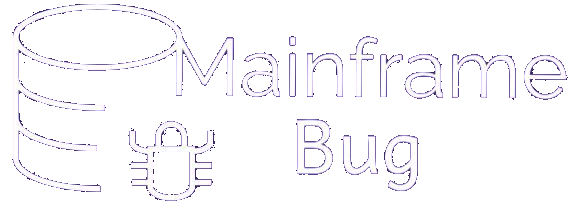## Module 6: Procedure Division

### COMPUTE verb

• In COBOL, Arithmetic expressions can be written using COMPUTE statement
• Operations performed using ADD, SUBTRACT, MULTIPLY and DIVIDE can also be performed using COMPUTE statement
• It is powerful verb because it allows to specify combined arithmetic operation
• Basic syntax:-

COMPUTE identifier-1 [ROUNDED] [, identifier-2 [ROUNDED]]…       = <arithmetic-expression>       [ON SIZE ERROR  <imperative-statement> ]

• Resultant value of arithmetic-expression will be stored in identifier-1, identifier-2 etc.
• ROUNDED, ON SIZE ERROR option can be used with COMPUTE. (Please refer ROUNDED , ON SIZE ERROR modules for more information)
• All identifiers participating in COMPUTE must be numeric items
• Literals are allowed in arithmetic-expression
• The sequence of operations in an arithmetic expression is as given below:-
The rule is left to right within the same order
Sequence Operation
1 Brackets ( )
2 NOT and Exponentiation (**)
3 AND, Multiplication(*) and Division (/)
4 OR, Addition (+) and subtraction (-)

Examples:-

Valid expressions using COMPUTE:-

1. COMPUTE A = 4 * I
2. COMPUTE TOTAL = RATE * QTY – DISCOUNT
Here, first RATE will be multiplied by QTY and then resulted product will be subtracted by DISCOUNT
3. COMPUTE Z = A - ( B + C ) * D ** 5
Meaning, Z = A – (B+C) * D5 and expression will be solved based on order provided in above table
4. COMPUTE AVERAGE = (SUB1-MARKS + SUB2-MARKS) / 2
5. COMPUTE FAHRENHEIT = 9/5 * CELSUIS +32
6. COMPUTE C = A**2 + B**2
Meaning C = A2 + B2
7. COMPUTE A = -B

Some Invalid expressions (which is not allowed while using COMPUTE):-

1. COMPUTE C = A+ -B
This COMPUTE statement is invalid because + and – cannot appear together side by side
2. COMPUTE Y = 3(A-1)
This COMPUTE statement is invalid because no operator coded between literal ‘3’ and expression (A-1)